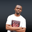Related Tags

php
web development
how to
communitycreator

# How to convert datetime in PHP to a Unix timestampNDUKWE CHIDERA K.

### Overview

While working with the MySQL database in PHP, we may need to record the time instance at which an event occurs. This instance can be stored as a table record. We can the instance as a table record by using the datetime or timestamp MySQL data types. The problem associated with this procedure is that it cannot display the date in a very human-friendly and easily-readable format.

We get the following from the datetime and timestamp MySQL table columns:

2021-11-17 13:02:18

But we may want to display the date in the format shown in the image below:

A better, more common date format

This shot teaches us how to display a date in this format, as a PHP script.

Before we learn to display the date in the aforementioned format, let’s look at the problems associated with MySQL table timestamp columns.

### Problems

• The datetime from the table is a string.
• It comes from the database table column of type timestamp, which usually presents datetime in the format 2021-11-17 13:02:18.
• This format is not a Unix timestamp. We’ll need to convert the format to a Unix timestamp in order to get the date in the format shown in image above.

### Converting datetime strings to a Unix timestamp

A combination of two PHP functions, the strtotime() and the date() functions, will produce an output like the one shown in the image above.

• The strtotime() function converts any given date string into a Unix timestamp. It only accepts the date string as a parameter.
• The date() function outputs the current time, or the time relative to the provided timestamp (if any), in the specified format. It takes the format we wish to display the date in and the optional timestamp as its two parameters.

Now, we will delve into some code examples.

### The incorrect approach

The code below is an incorrect way of trying to get the output we’ve shown above, which may be our first approach while trying out solutions to that problem for the first time.

The output is not what we want, and that is because we didn’t convert the timestamp into a proper Unix timestamp before passing it to the date() function.

<?php
$mytimestamp = '2021-11-17 13:02:18'; //$dating = intval($mytimestamp); // echo$dating;
$converted = date('m d, Y',$mytimestamp);
echo $converted; ?> How not to convert timestamps ### The correct approach In this second code, we use the strtotime() function to convert the datetime string from a database table into a Unix timestamp. Then, with the help of the date() function, we set the timestamp to various date formats of our choice. ### What is the Unix timestamp? This is the number of seconds since the Unix epoch time (01-01-1970). For more tips on the PHP date() function, we can see this post. Note: The escape character is used in the code snippet below to escape the words that are just used as text, so that they are not given special interpretation by the function. ### Code explanation In the code below, a datetime string, saved in the variable $d, is converted to a Unix timestamp with the strtotime() function. It is then saved to the variable $ad on line 5. <?php //saving my database fetched datetime in a variable$d = '2021-11-17 13:02:18';
//force my db datetime into unix timestamp
$ad = strtotime($d);
//see what $ad variable value looks like echo$ad."\n";
//use $ad the timestamp to get date$realdate = date('M d, Y',$ad); //get another fancy date$real = date('l F jS, 2021',$ad); //a more fancy one$realfancy = date('\t\h\i\s \i\s \t\h\e zS \d\a\y, \i\n Y',$ad); //let add time to it$realdateTime = date('\O\n: dS M, Y \B\y: ga',$ad); //the date now looks like what I initially wanted echo$realdate ."\n";
echo $real ."\n"; echo$realfancy ."\n";
echo $realdateTime ."\n"; ?> The correct approach With the help of the date() function and the value of $ad, a timestamp is used to set and show dates and time in different formats.

RELATED TAGS

php
web development
how to
communitycreator

CONTRIBUTORNDUKWE CHIDERA K.
RELATED COURSES

View all Courses

Keep Exploring

Learn in-demand tech skills in half the time